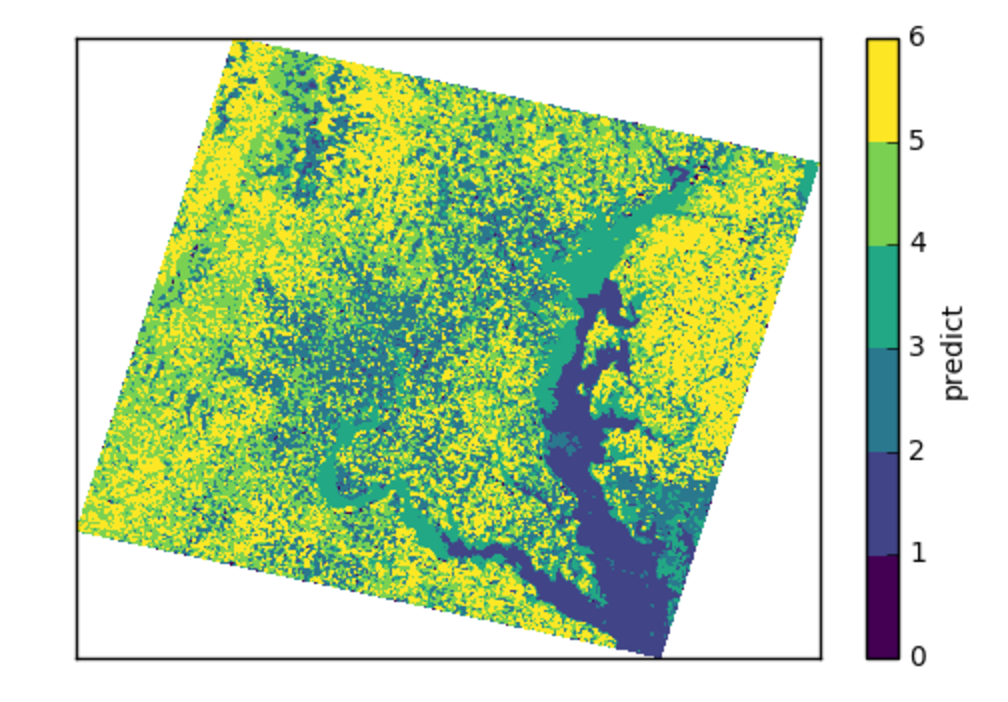# Tutorials¶

This page walks through a `Jupyter` notebook using `elm` to ensemble fit K-Means and predict from all members of the ensemble.

It demonstrates the common steps of using `elm` :

• Working with `earthio.load_array` to read `NetCDF` , `HDF4` , `HDF5` , and GeoTiff files, and controlling how a sample is composed of bands or separate rasters with `LayerSpec` . See also Creating an ElmStore from File
• Defining a `Pipeline` of transformers (e.g. normalization and PCA) and an estimator, where the transformers use classes from `elm.pipeline.steps` and the estimator is a model with a `fit` / `predict` interface. See also Pipeline
• Calling fit_ensemble to train the Pipeline under varying parameters with one or more input samples
• Calling predict_many to predict from all trained ensemble members to one or more input samples

## LANDSAT¶

The LANDSAT classification is notebook from elm examples. This section walks through that notebook, pointing out:

• How to use `earthio` for scientific data files like GeoTiffs
• How to set up an `elm.pipeline.Pipeline` of transformations
• How to use `dask` to `fit` a `Pipeline` in ensemble and predict from many models

NOTE : To follow along, make sure you follow the Prerequisites for Examples. The LANDSAT sample used here can be found in the AWS S3 LANDSAT bucket here.

## `earthio` Walk-Through¶

First the notebook sets some environment variables related to usage of a `dask-distributed` `Client` and the path to the GeoTiff example files from `elm-data`_:

Each GeoTiff file has 1 raster (band of LANDSAT data):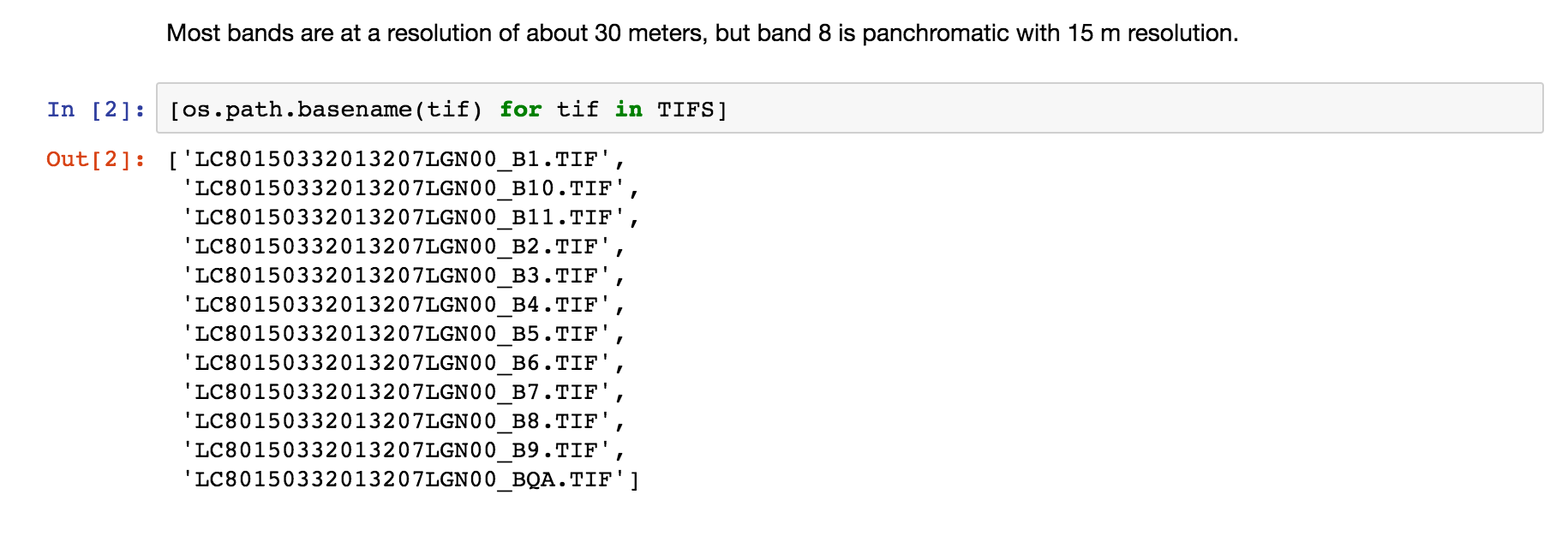See more inforation on `ElmStore` in ElmStore.

## `earthio.LayerSpec`¶

Using a list of `LayerSpec` objects, as shown below, is how one can control which bands, or individual GeoTiff files, become the sample dimensions for learning:

• `buf_xsize`: The size of the output raster horizontal dimension
• `buf_ysize`: The size of the output raster vertical dimension
• `name`: What do call the band in the `ElmStore` returned. For example `band_1` as a name will mean you can use `X.band_1` and find `band_1` as a key in `X.data_vars`.
• `search_key`: Where to look for the band identifiying info, in this case the file `name`
• `search_value` : What string token identifies a band, e.g. `B1.TIF` (see file names printed above)

We are using `buf_xsize` and `buf_ysize` below to downsample.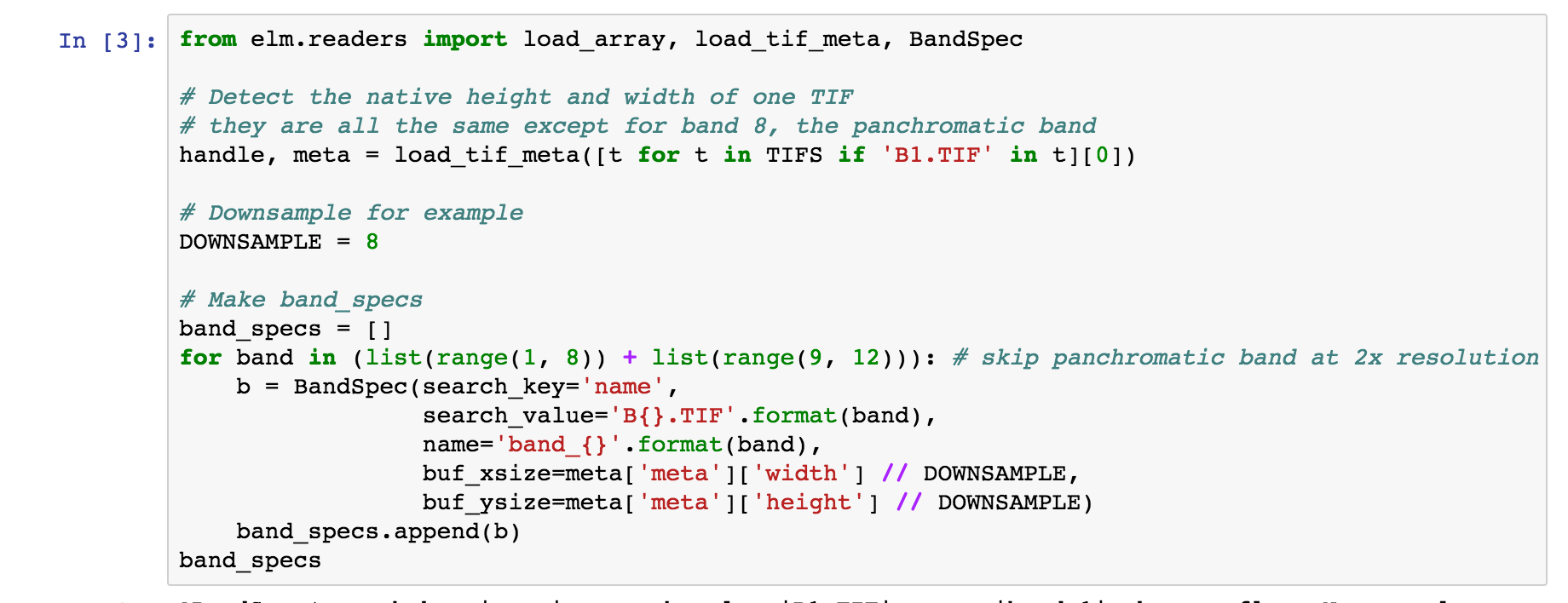Check the `repr` of the `LayerSpec` objects to see all possible arguments controlling reading of bands: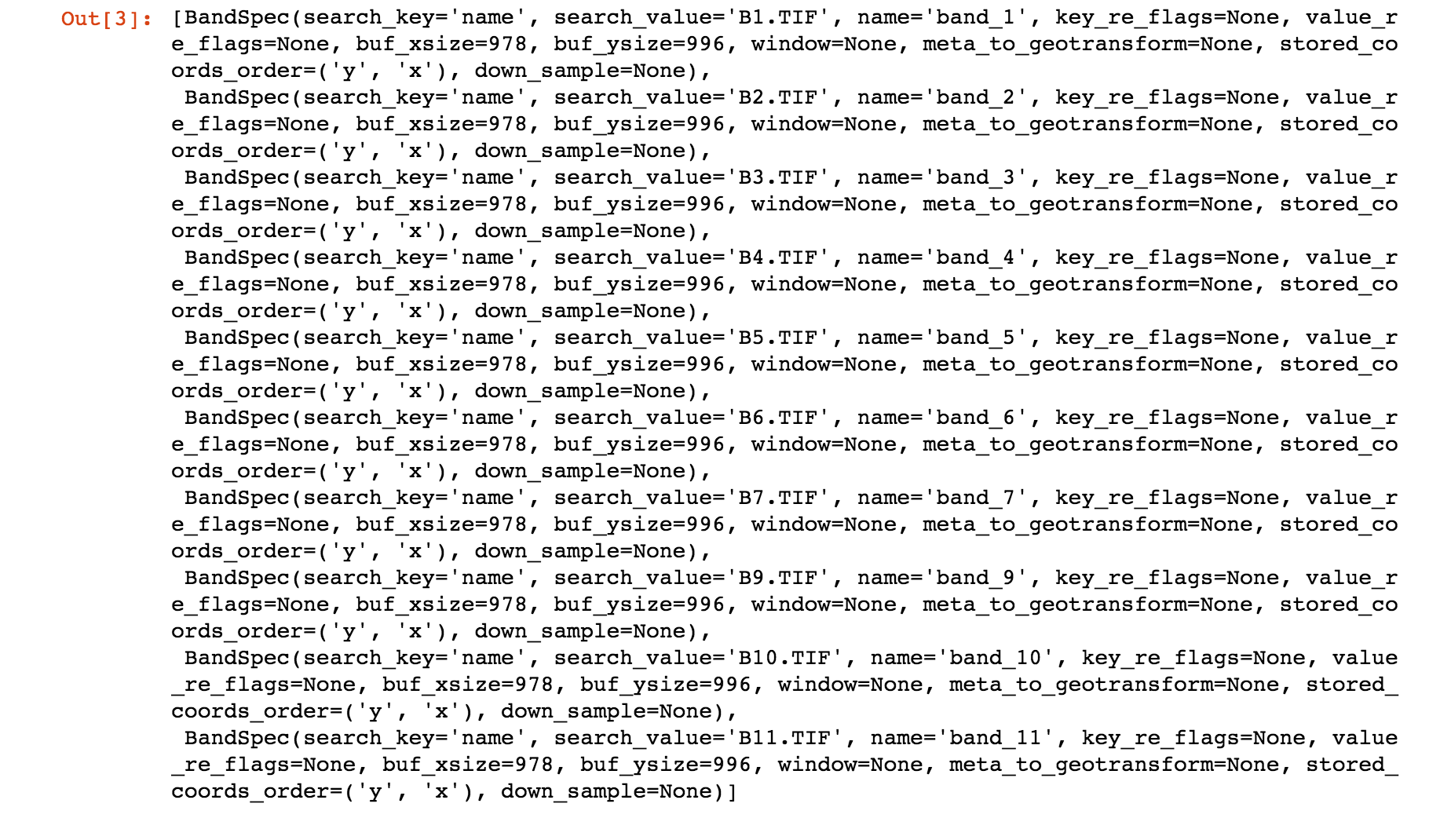## `earthio.load_array`¶

`load_array` aims to find a file reader for a `NetCDF`, `HDF4`, `HDF5`, or GeoTiff source.

The first argument to `load_array` is a directory if reading GeoTiff files and it is assumed that the directory contains GeoTiff files each with a 1-band raster.

For `NetCDF`, `HDF4`, and `HDF5` the first argument is a single filename, and the bands are taken from the `variables` (`NetCDF`) or `subdatasets` (`HDF4` / `HDF5`).

`band_specs` (list of `LayerSpec` objects) is passed in to `load_array` (the list of `LayerSpec` objects from above) to control which bands are read from the directory of GeoTiffs.## Using an `ElmStore` like an (xarray.Dataset)¶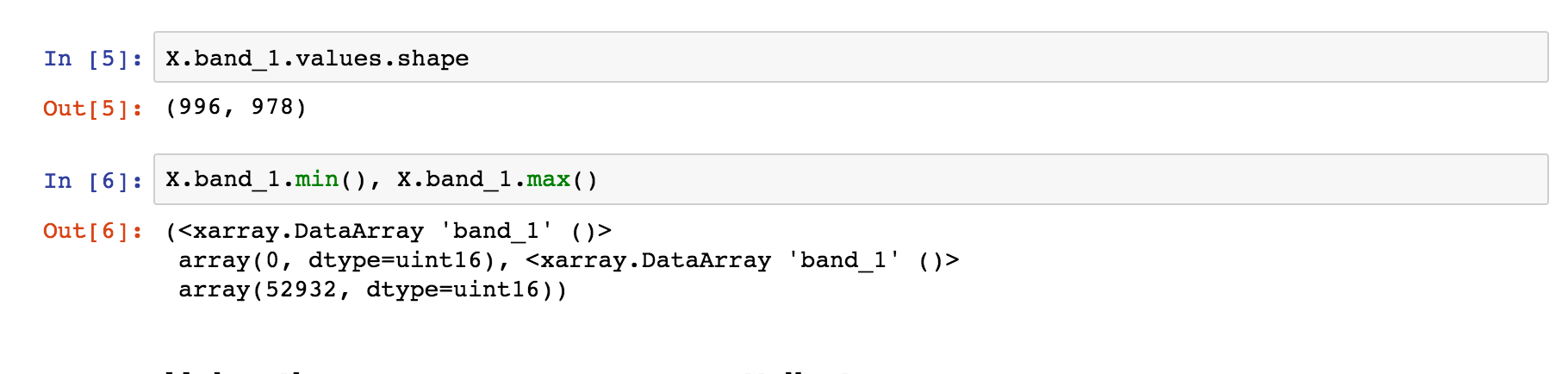## Visualization with `ElmStore`¶

The notebook then goes through a number of examples similar to:

• `X.band_1.plot.pcolormesh()` - The code uses names like `band_1`, `band_2`. These are named `DataArray` objects in the `ElmStore` `X` because of the `name` argument to the `LayerSpec` objects above. The `plot.pcolormesh()` comes from the data viz tools with xarray.DataArray .
• The output of `X.band_1.plot.pcolormesh()`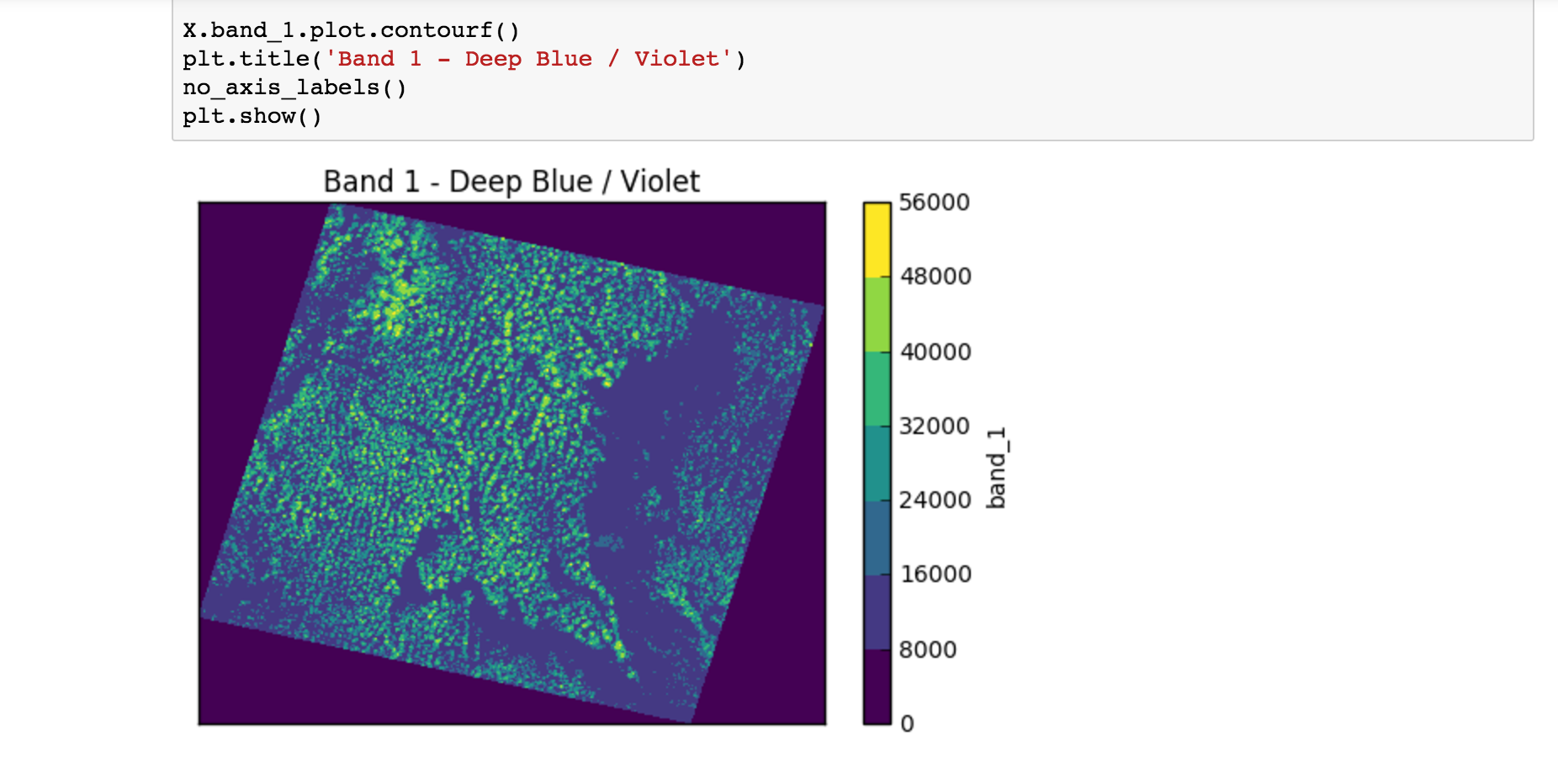## Building a `Pipeline`¶

Building an `elm.pipeline.Pipeline` of transformations is similar to the idea of a Pipeline in scikit-learn.

• All steps but the last step in a Pipeline must be instances of classes from the elm.pipeline.steps - these are the transformers.
• The final step in a Pipeline should be an estimator from scikit-learn with a fit/predict interface.
The notebook shows how to specify a several-step Pipeline of
• Flattening rasters
• Assigning NaN where needed
• Dropping NaN rows
• Standardizing (Z-scoring) by band means and standard deviations
• Adding polynomial interaction terms of degree two
• Transforming with PCA
• K-Means with partial_fit several times per model

Preamble - Imports

This cell show typical import statments for working with a `elm.pipeline.steps` that become part of a `Pipeline`, including importing a transformer and estimator from scikit-learn: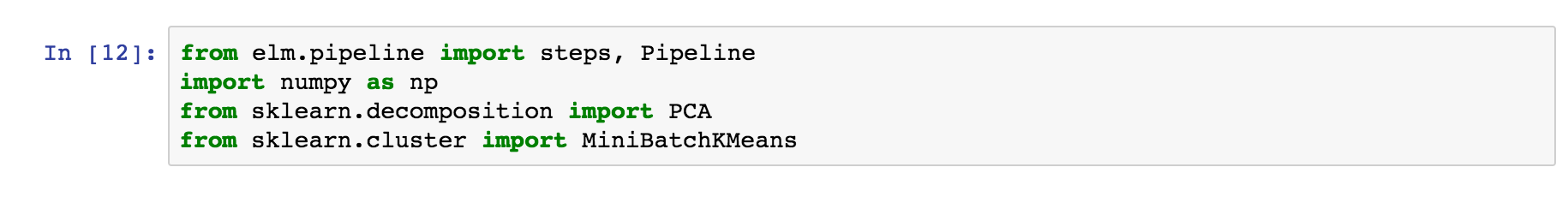## Steps - `Flatten`¶

This transform-flatten step is essentially `.ravel` on each `DataArray` in `X` to create a single 2-D `DataArray` :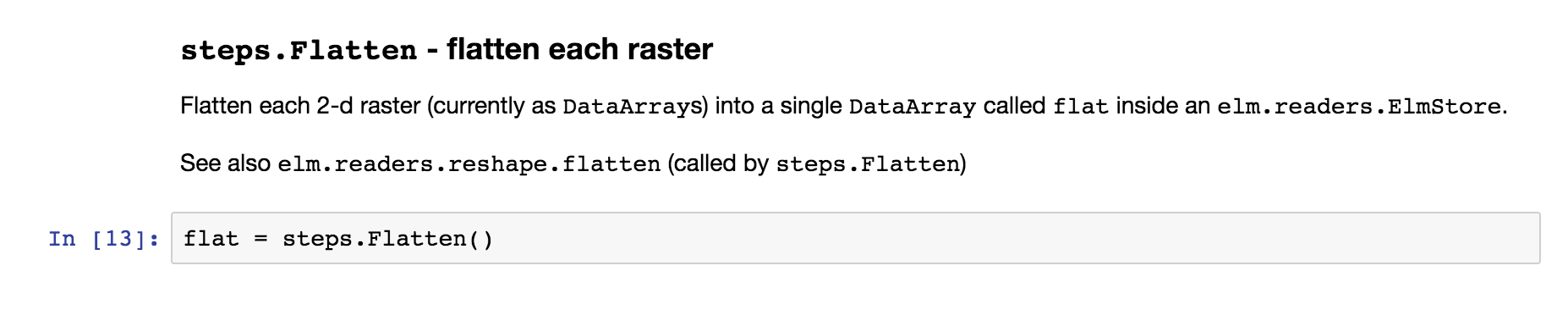## Steps - `ModifySample` - `set_nans`¶

The next step uses `elm.pipeline.steps.ModifySample` to run a custom callable in a `Pipeline` of transformations. This function sets `NaN` for the no-data perimeters of the rasters: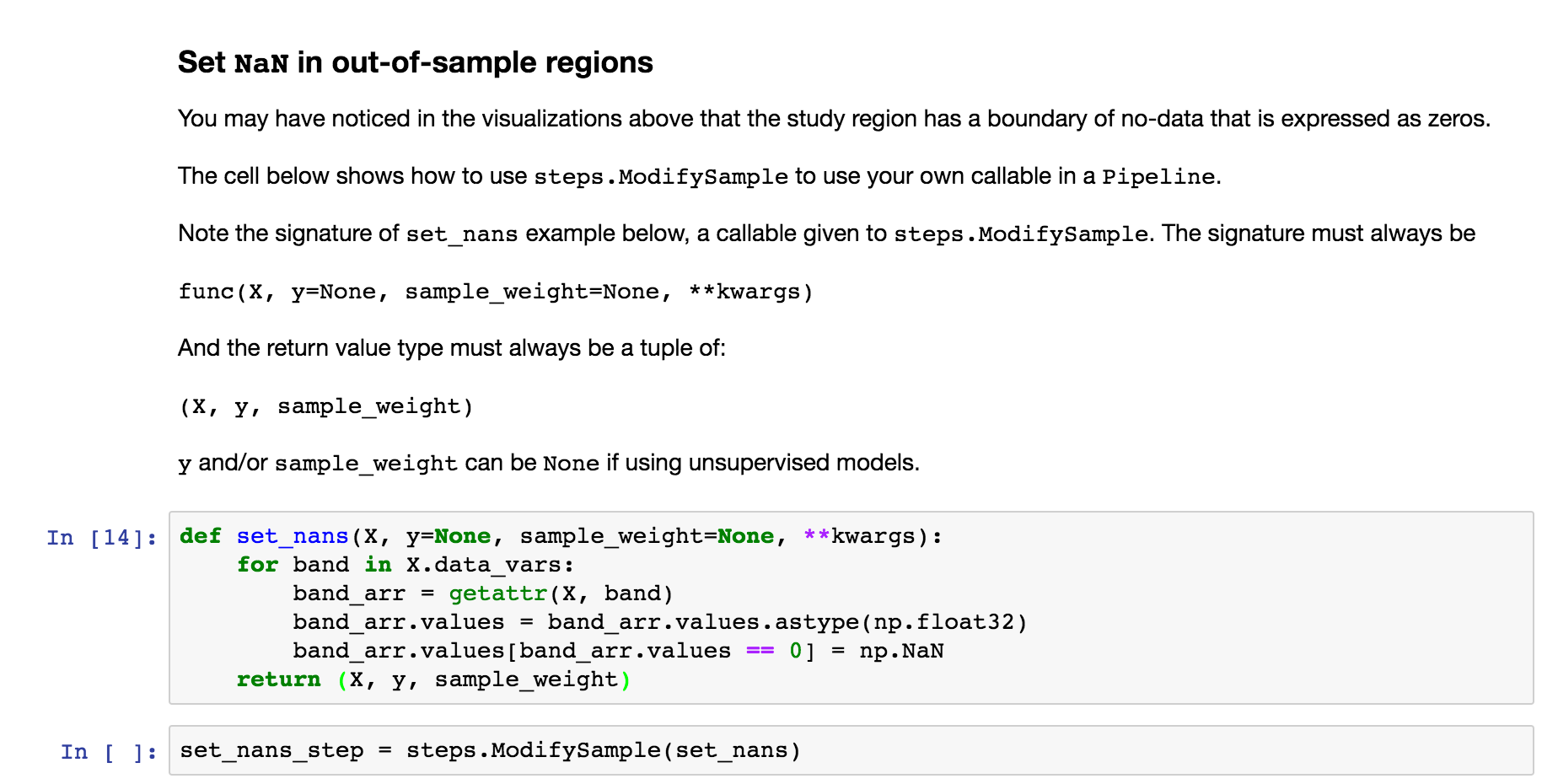## Steps - `DropNaRows` - Drop Null / NaN Rows¶

The transform-dropnarows is a transformer to remove the `NaN` values from the `DataArray` `flat` (the flattened (`ravel`) rasters as a single 2-D `DataArray` )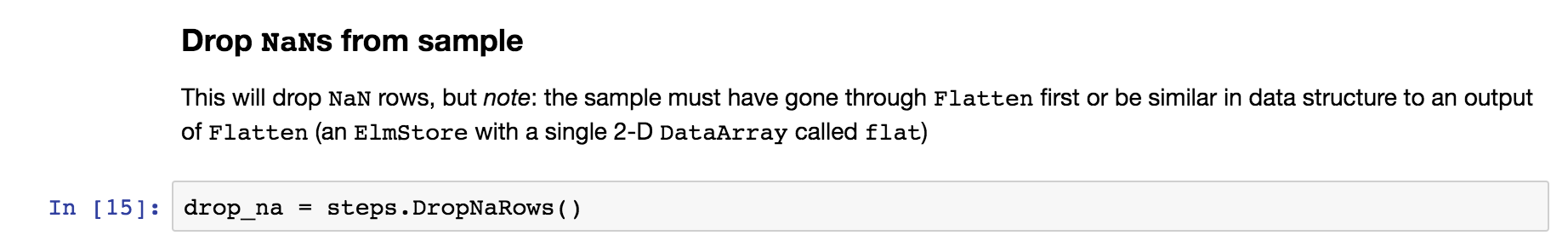## Steps - `ModifySample` - Log Transform (or pass through)¶

This usage of `ModifySample` will allow the `Pipeline` to use log transformation or not (see usage of `set_params` several screenshots later)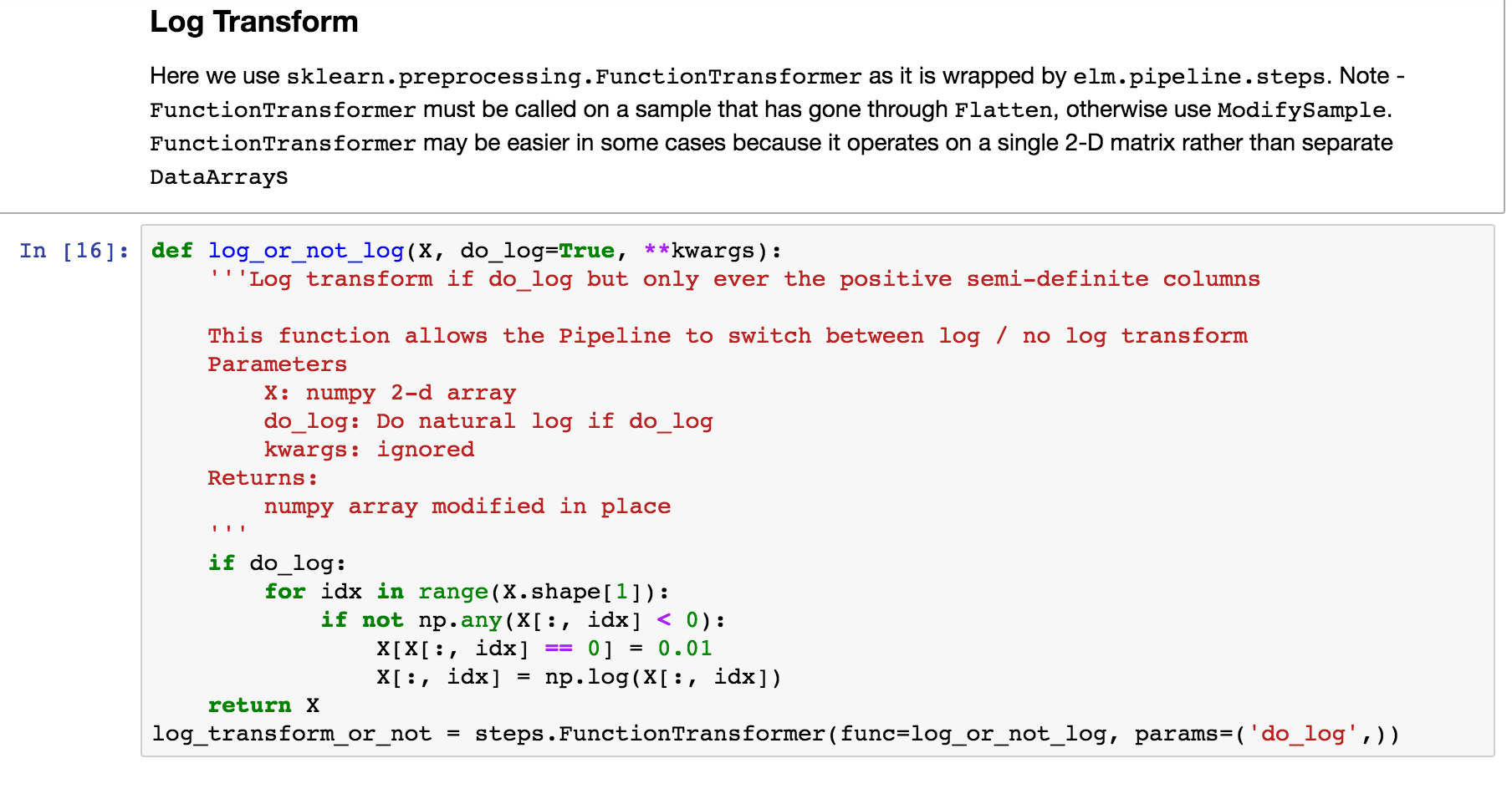## Feature engineering in a `Pipeline`¶

Define a function that can do normalized differences between bands (raster or `DataArray` ), adding the normalized differences to what will be the `X` data in the `Pipeline` of transformations.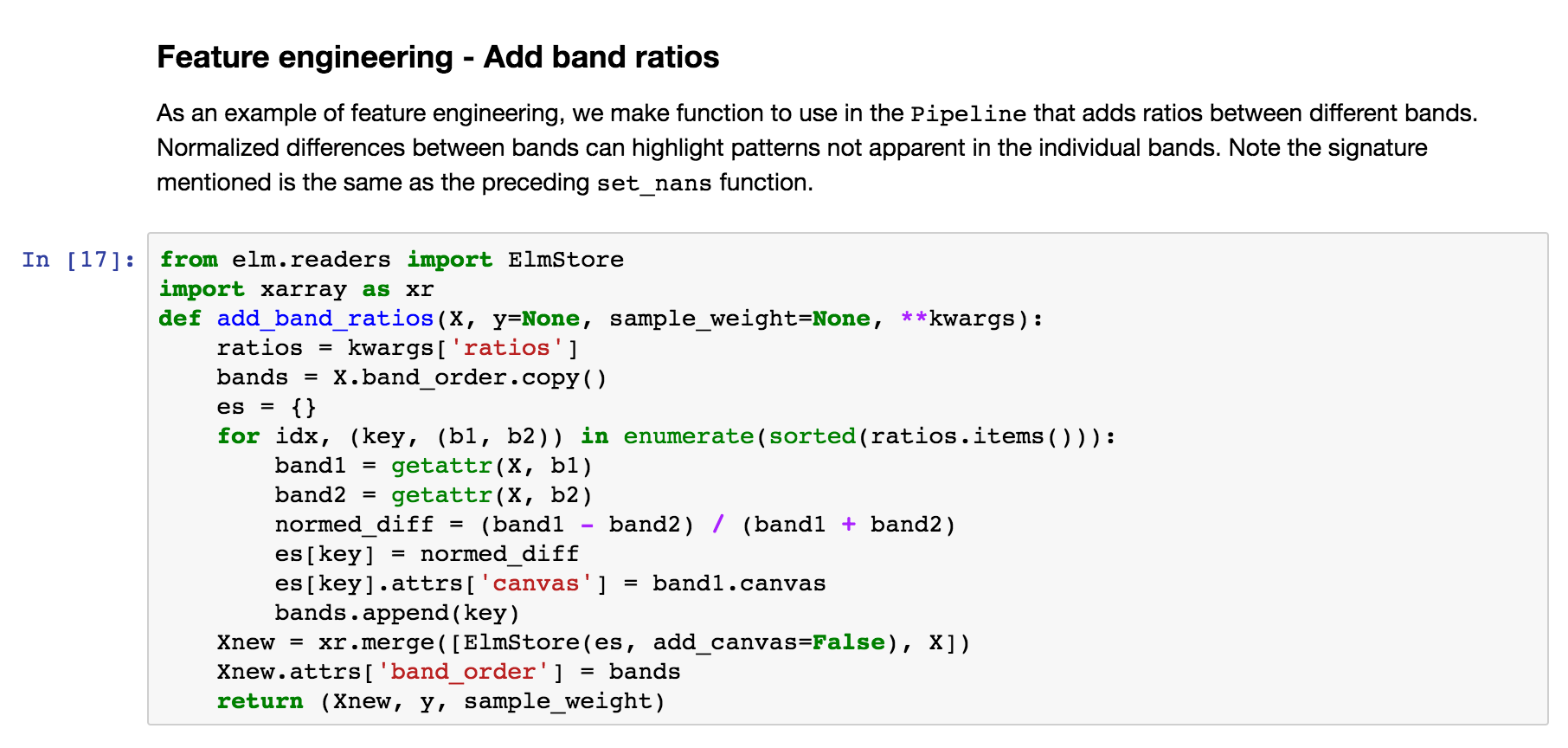## Feature engineering - `ModifySample` with arguments¶

And here is how the function above can be used in a `Pipeline` (wrapping with `elm.pipeline.steps.ModifySample` ):

We are calculating:

• `NDWI` : Normalized Difference Water Index * `(band_4 - band_5) / (band_4 + band_5)`
• `NDVI` : Normalized Difference Vegetation Index * `(band_5 - band_4) / (band_5 + band_4)`
• `NDSI` : Normalized Difference SnowIndex * `(band_2 - band_6) / (band_2 + band_6)`
• `NBR` : Normalized Burn Ratio * `(band_4 - band_7) / (band_7 + band_4)`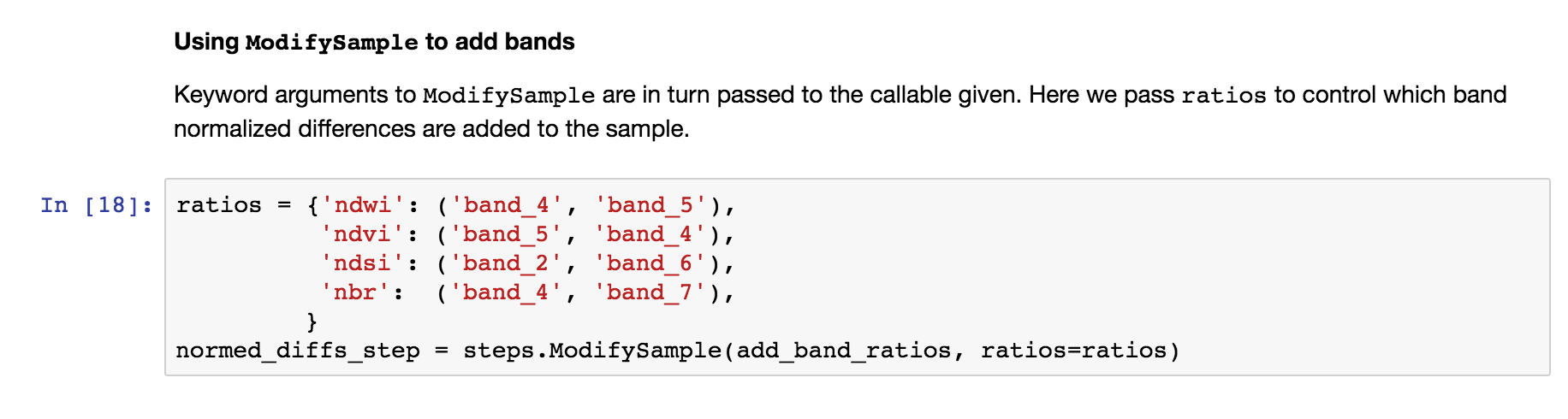Using pcolormesh on normalized differences of bands

Here are the `NDWI` and `NDVI` plotted with the `xarray-pcolormesh`_ method of the `predict` `DataArray`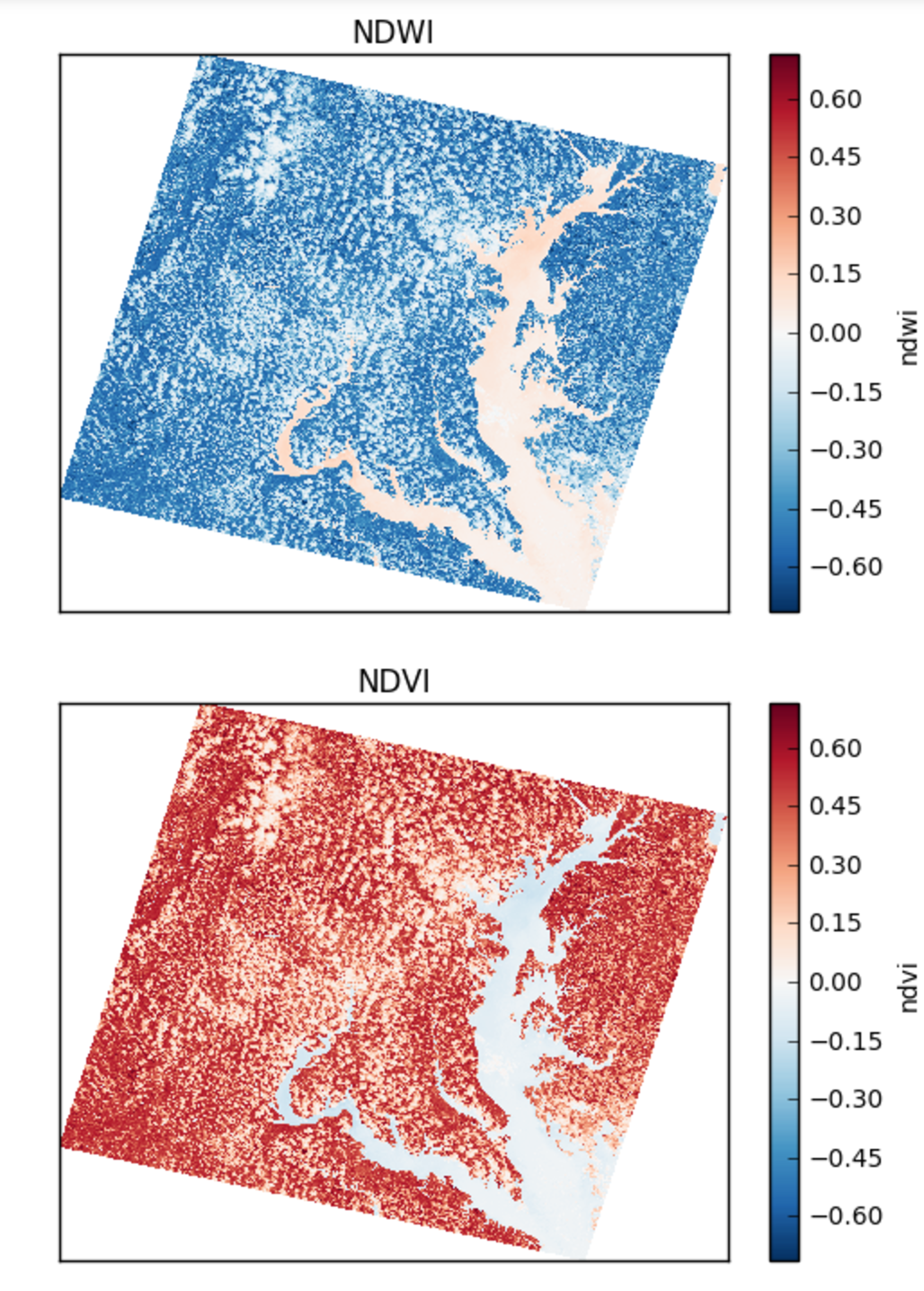False Color with normalized differences of bands

The image below has an RGB (red, green, blue) matrix made up of the `NBR` , `NDSI` , `NDWI` normalized differences: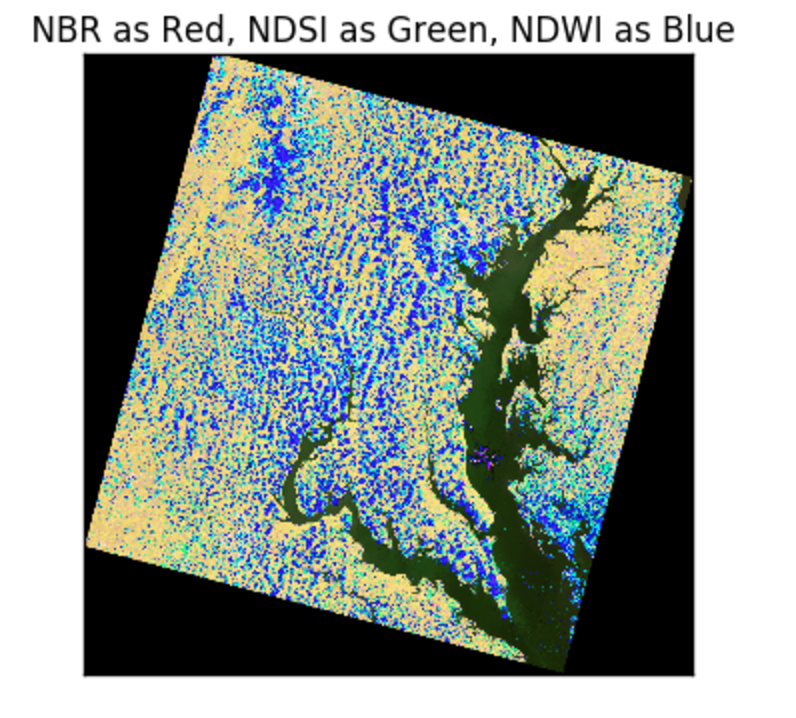## Normalization and Adding Polynomial Terms¶

The following snippets show how to use a class from `sklearn.preprocessing` or `sklearn.feature_selection` with `Pipeline` :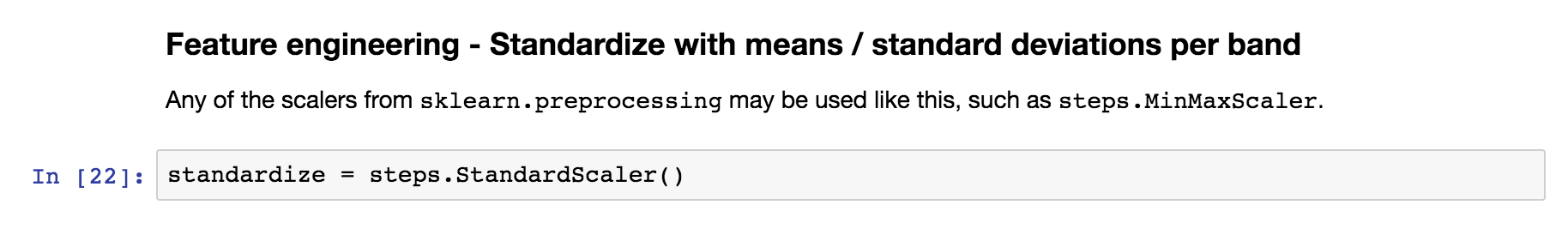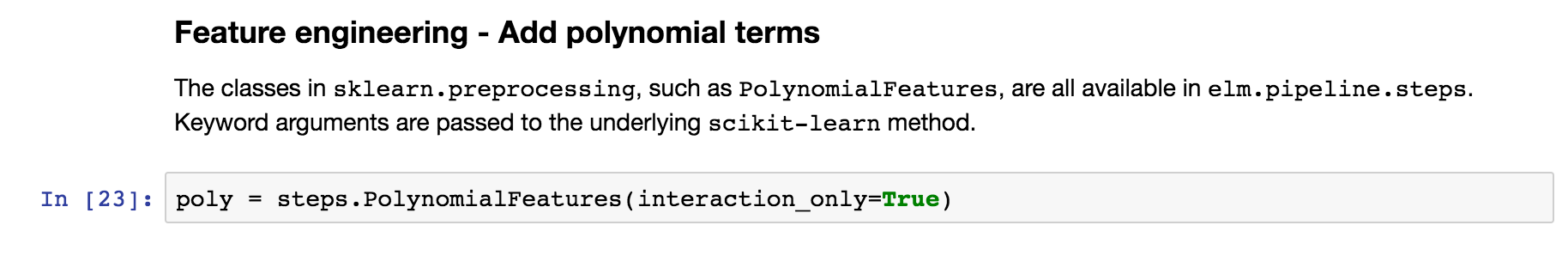Custom Feature Selection

By defining the function below, we will be able to choose among random combinations of the original data or normalized differences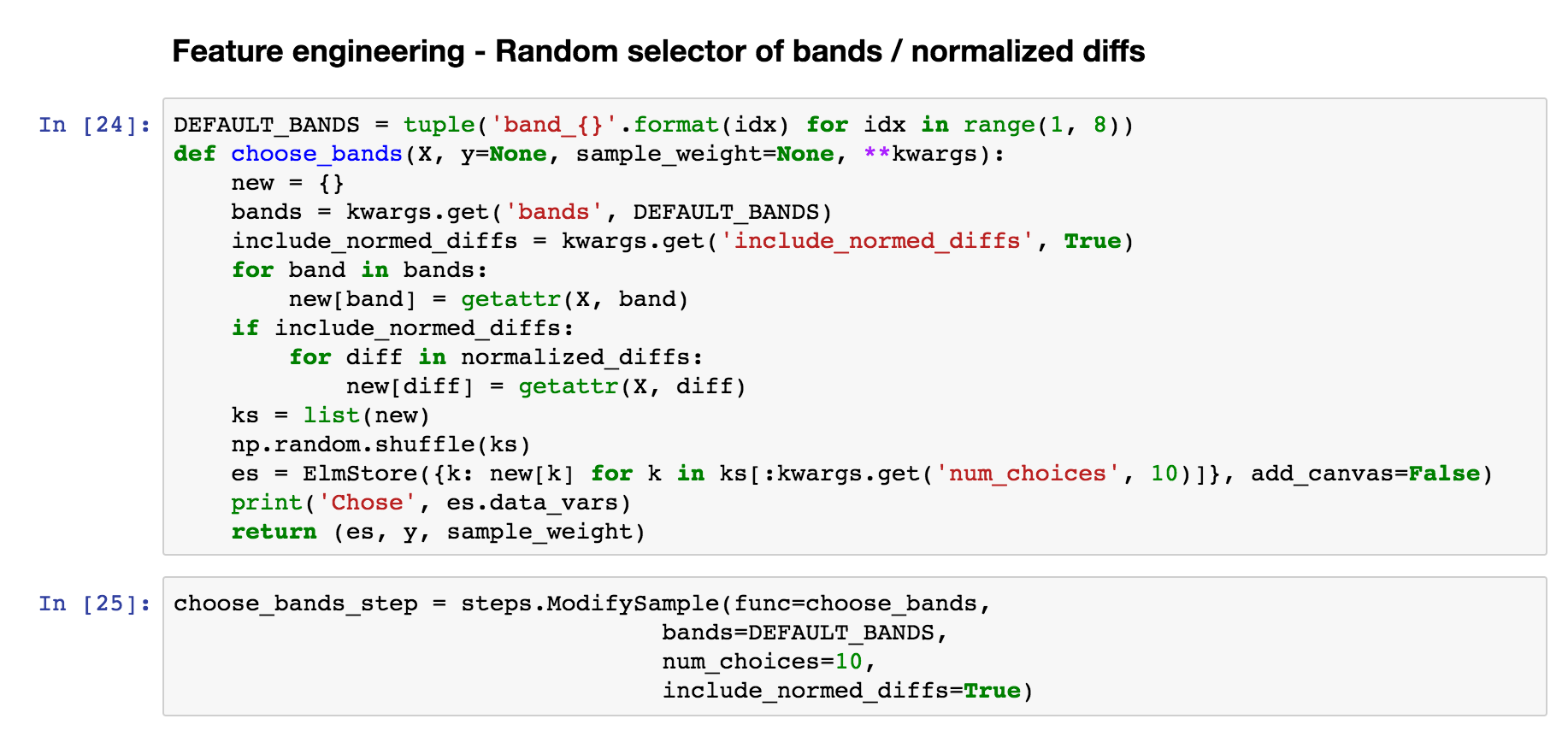## PCA¶

Use `steps.Transform` to wrap `PCA` or another method from `sklearn.decomposition` for `elm.pipeline.Pipeline` .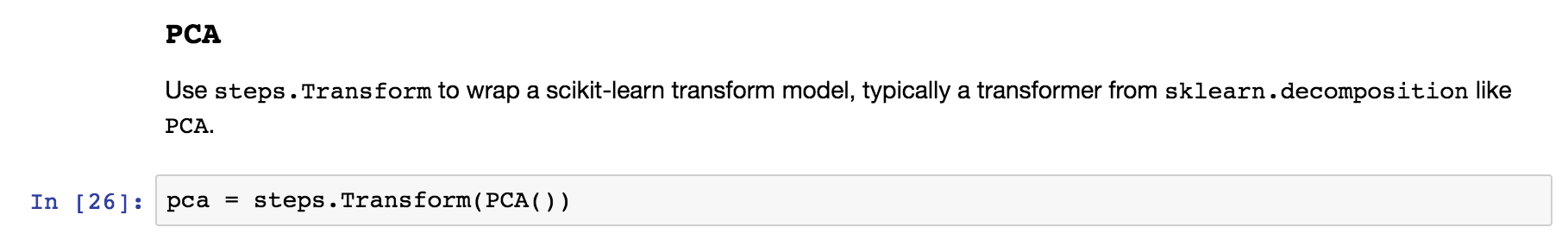## Use an estimator from `scikit-learn`¶

Use a model with a `fit` / `predict` interface, such as `KMeans`.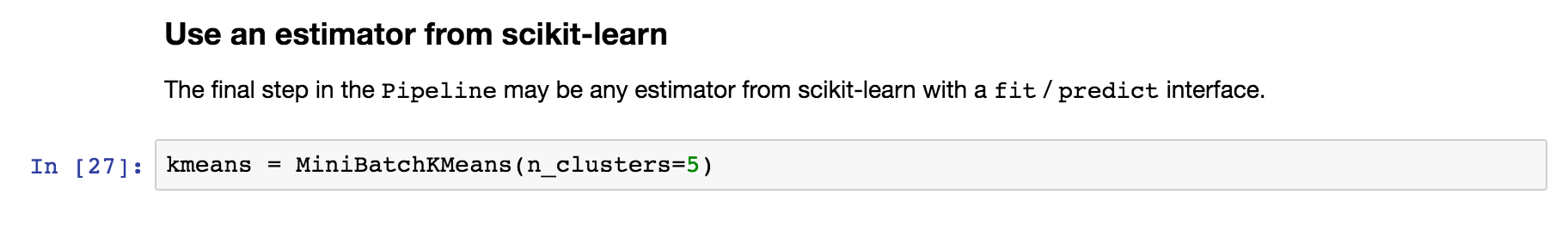Most scikit-learn models described here are supported.

## Create `Pipeline` instance¶

The following uses all the steps we have created in sequence of tuples and configures scoring for K-Means with the Akaike Information Criterion.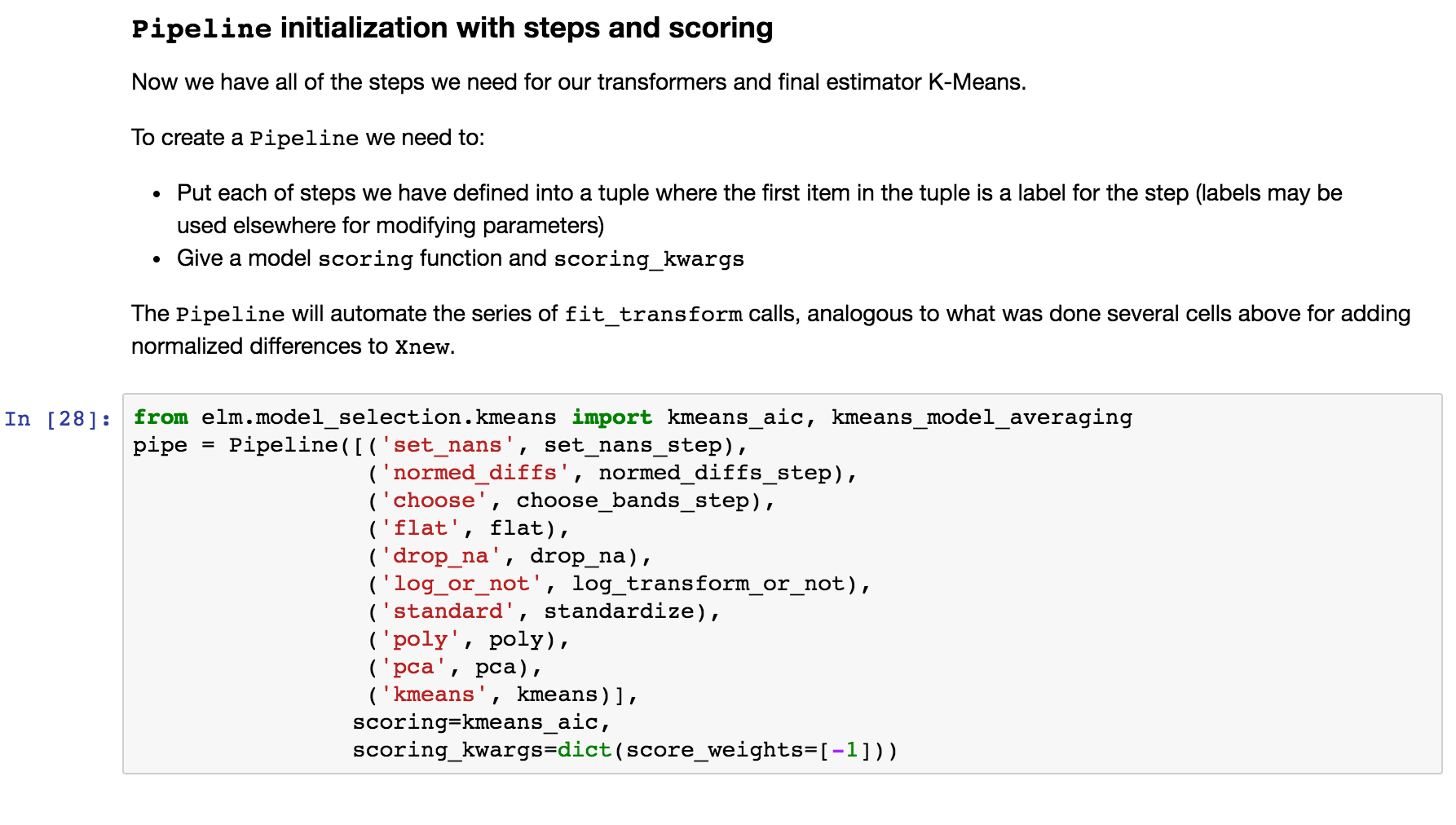The next steps deal with controlling fit_ensemble (fitting with a group of models of different parameters)

## `ensemble_init_func`¶

This is an example `ensemble_init_func` to pass to fit_ensemble, using `pipe.new_with_params(**new_params)` to create a new unfitted `Pipeline` instance with new parameters.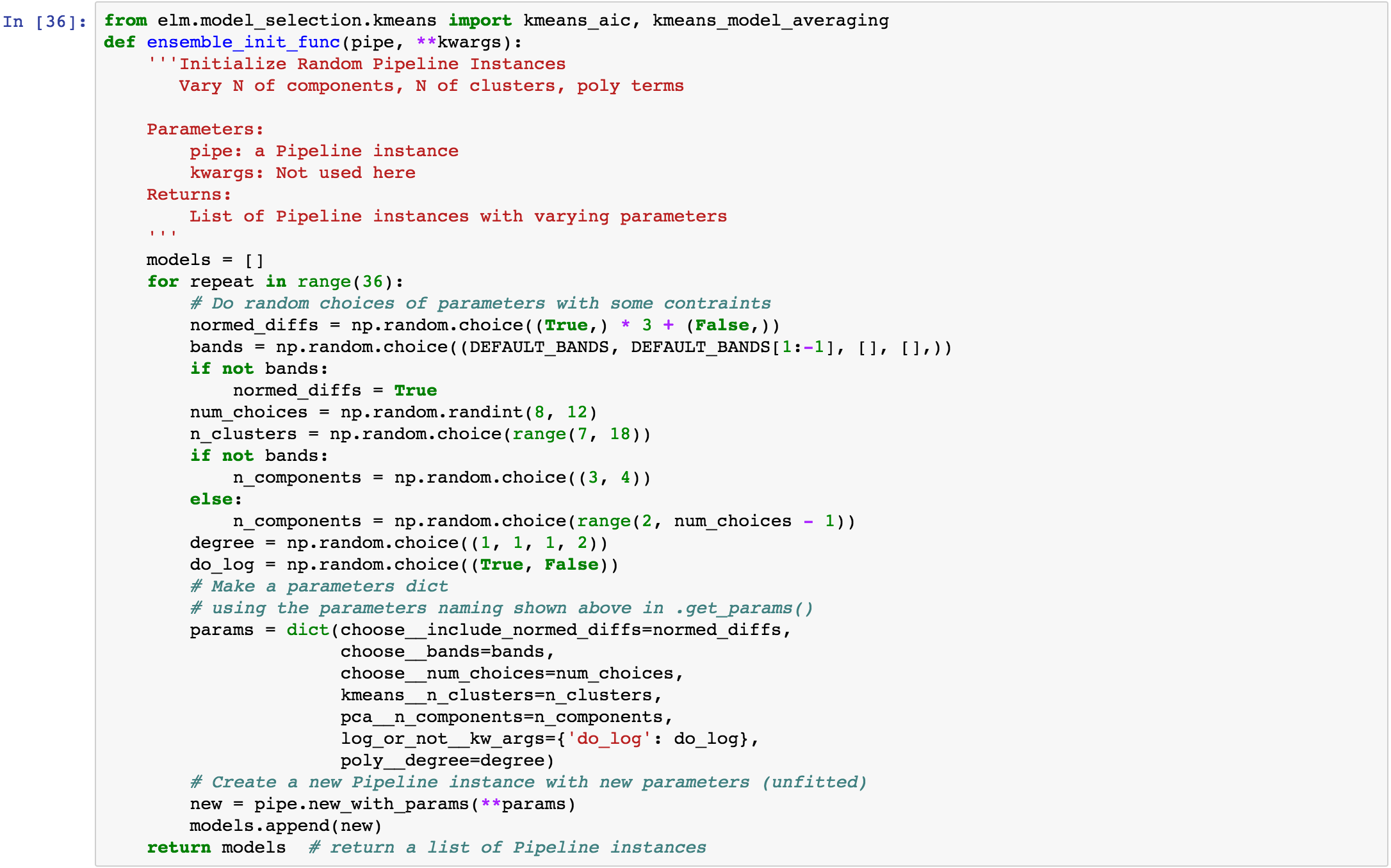The fit_ensemble docs also show an example of an `ensemble_init_func`.

## More `fit_ensemble` control¶

The following sets the number of generations ( `ngen` ) and the `model_selection` callable after each generation.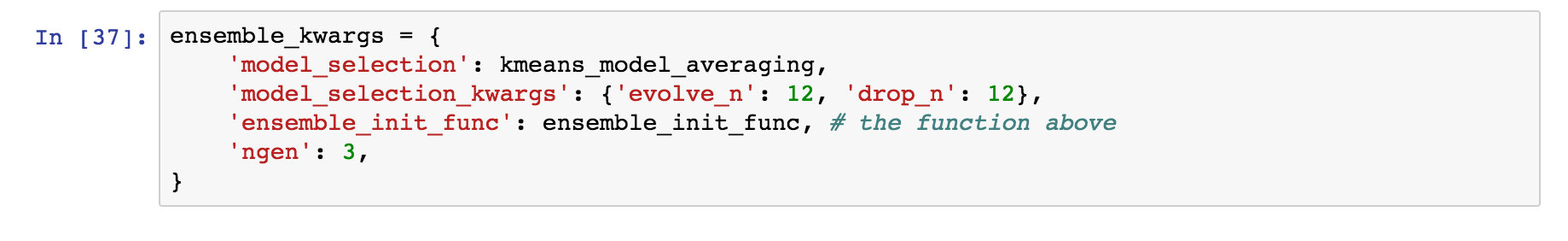## Parallelism with `dask-distributed`¶

fit_ensemble , to fit a group of models in generations with model selection after each generation, and predict_many each take a `client` keyword as a dask `Client` (dask). predict_many parallelizes over multiple models and samples, though here only one sample is used.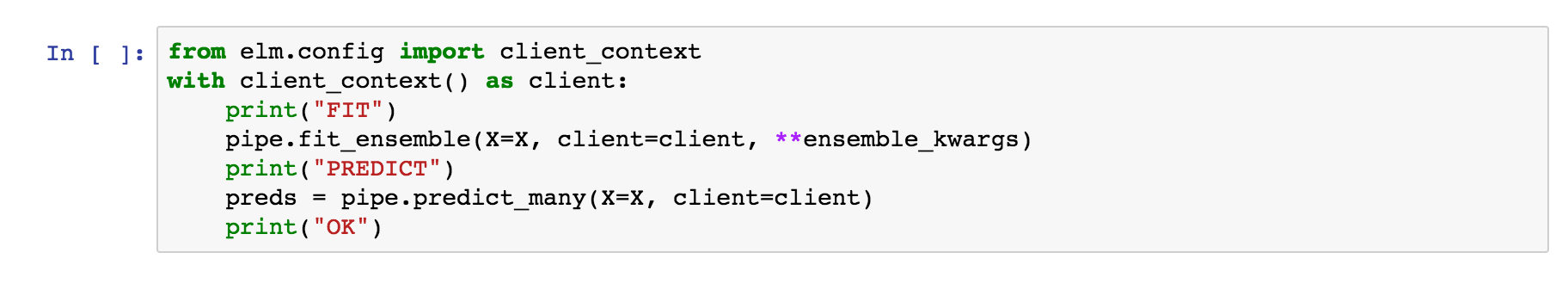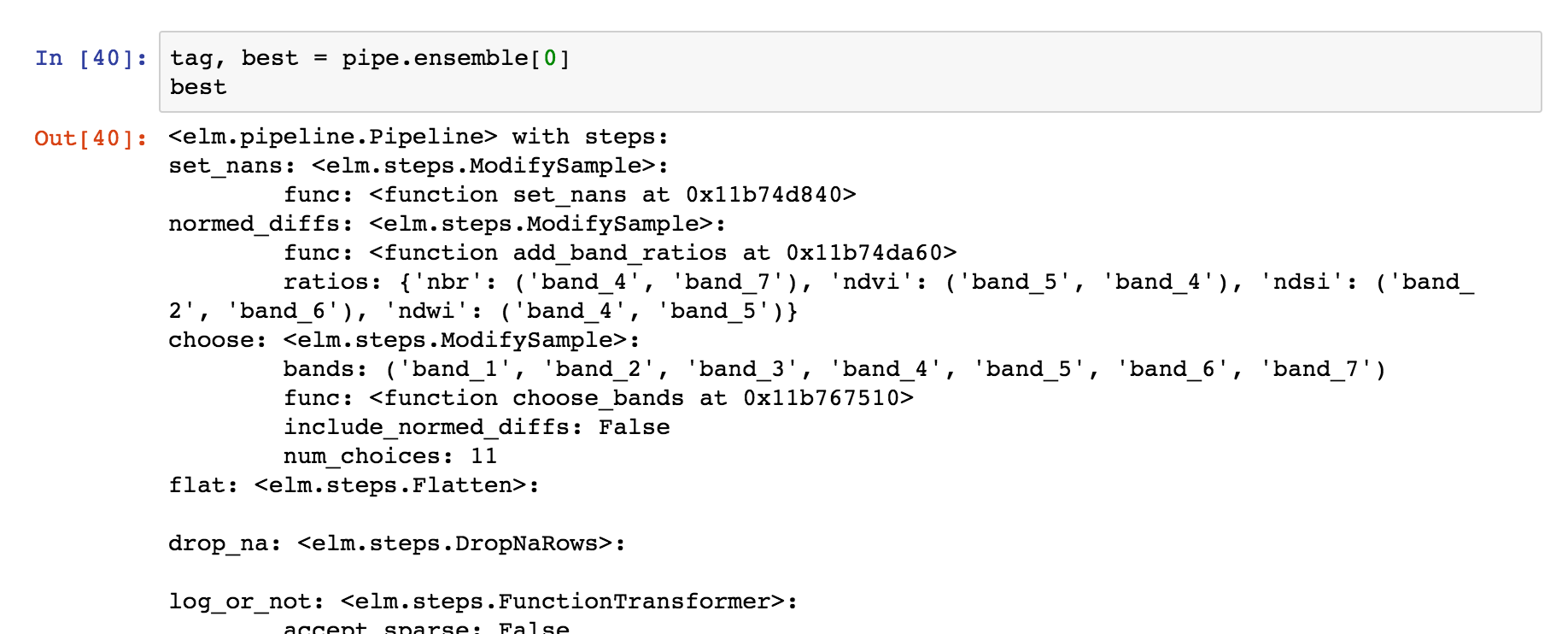## Using an `ElmStore` from predict_many¶

predict_many has called transform-inverseflatten to reshape the 1-D numpy array from the `sklearn.cluster.KMeans.predict` method to a 2-D raster with the coordinates of the original data. Note also the `inverse_flatten` is typically able to preserve `NaN` regions of the original data (the `NaN` borders of this image are preserved).

Using the xarray’s pcolormesh on the `predict` attribute ( `DataArray` ) of an `ElmStore` returned by predict_many :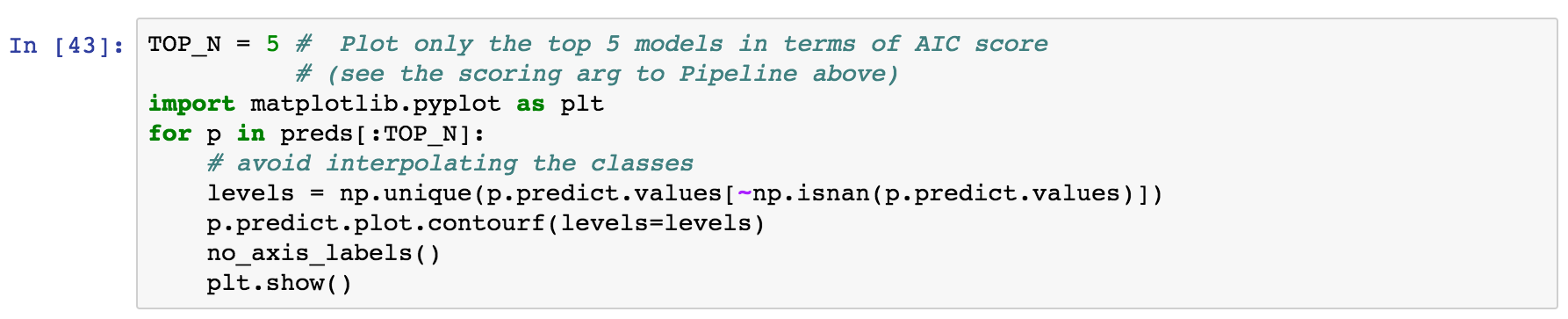The best prediction in terms of `AIC` :